### Revenge of the Baby-SatHover over the thumbnail for a full-size version.

Author Jawbit author:jawbit calvin n-art rated 2009-09-21 2009-09-21 5 by 41 people. \$Revenge of the Baby-Sat#Jawbit#n-art#00000000000000000000000000000000000000000000000000000000000000000000000000000000000000000000000000000000000000000000000000000000000000000000000000000000000000000000000000000000000000000000000000000000000000000000000000000000000000000000000000000000000000000000000000000000000000000000000000000000000000000000000000000000000000000000000000000000000000000000000000000000000000000000000000000000000000000000000000000000000000000000000000000000000000000000000000000000000000000000000000000000000000000000000000000000000000000000000000000000000000000000000000000000000000000000000000000000000000000000000000000000000000000000000000000000000000000000000000000000000000000000000000000000000000000000000000000000000000000|12^330,374!12^328,370!12^322,358!12^315,354!12^309,352!12^304,350!12^298,344!12^295,337!12^291,332!12^290,325!12^286,323!12^279,322!12^276,324!12^272,325!12^267,326!12^265,328!12^261,328!12^254,327!12^250,324!12^245,320!12^241,316!12^238,309!12^234,303!12^231,297!12^229,291!12^228,284!12^231,278!12^234,272!12^239,270!12^246,268!12^249,266!12^253,268!12^257,270!12^262,273!12^265,274!12^271,274!12^274,274!12^275,270!12^273,266!12^270,262!12^269,257!12^268,253!12^267,249!12^266,245!12^262,240!12^260,237!12^257,231!12^253,231!12^249,231!12^245,232!12^242,232!12^238,232!12^231,230!12^227,230!12^224,230!12^215,207!12^215,211!12^218,214!12^223,216!12^229,219!12^236,220!12^241,220!12^245,220!12^248,220!12^251,220!12^254,220!12^259,220!12^264,220!12^266,216!12^263,213!12^258,208!12^252,204!12^248,197!12^243,189!12^239,184!12^237,180!12^251,202!12^241,188!12^266,196!12^262,190!12^260,185!12^257,181!12^255,176!12^251,170!12^248,163!12^246,160!12^284,178!12^283,171!12^283,164!12^283,156!12^283,151!12^287,145!12^287,138!12^288,132!12^289,124!12^290,118!12^292,109!12^293,104!12^325,135!12^328,126!12^331,118!12^334,111!12^337,104!12^338,99!12^342,93!12^347,85!12^350,80!12^354,73!12^357,66!12^362,59!12^365,54!12^370,47!12^373,41!12^376,39!12^380,37!12^387,37!12^389,41!12^389,46!12^389,53!12^389,57!12^388,65!12^388,61!12^388,68!12^386,72!12^384,80!12^384,85!12^384,98!12^383,108!12^383,113!12^383,104!12^384,113!12^392,108!12^400,106!12^405,103!12^413,99!12^410,100!12^420,95!12^435,86!12^441,81!12^448,79!12^455,77!12^458,74!12^463,72!12^469,69!12^475,65!12^481,60!12^491,56!12^499,51!12^504,47!12^510,48!12^512,50!12^515,57!12^500,49!12^495,55!12^277,248!12^280,247!12^282,247!12^278,235!12^281,235!12^344,169!12^347,167!12^353,166!12^355,164!12^373,170!12^377,168!12^383,167!12^387,164!12^392,163!12^399,163!12^405,163!12^410,163!12^416,163!12^426,163!12^422,163!12^431,163!12^438,163!12^443,163!12^449,164!12^456,164!12^462,167!12^346,238!12^351,235!12^355,229!12^354,232!12^360,227!12^367,222!12^363,225!12^371,220!12^376,220!12^385,220!12^391,222!12^396,226!12^403,226!12^408,229!12^413,229!12^417,229!12^380,220!12^422,227!12^426,224!12^431,219!12^433,216!12^438,210!12^440,207!12^442,203!12^446,199!12^450,194!12^454,191!12^460,190!12^466,188!12^474,188!12^480,188!12^485,190!12^511,65!12^507,68!12^506,74!12^513,62!12^502,78!12^498,83!12^497,88!12^494,93!12^492,97!12^489,101!12^487,104!12^484,107!12^481,112!12^480,117!12^480,122!12^480,127!12^480,128!12^484,128!12^491,128!12^484,111!12^498,126!12^502,125!12^509,125!12^517,123!12^524,123!12^531,123!12^536,123!12^542,121!12^547,119!12^554,116!12^560,115!12^565,117!12^566,117!12^572,125!12^570,128!12^565,128!12^562,131!12^555,136!12^550,139!12^546,141!12^542,145!12^537,151!12^535,154!12^532,159!12^529,162!12^536,162!12^543,163!12^547,163!12^551,162!12^555,162!12^560,162!12^565,162!12^571,162!12^576,162!12^582,162!12^588,162!12^592,162!12^594,162!12^542,186!12^551,186!12^557,188!12^562,190!12^568,191!12^571,191!12^575,192!12^582,195!12^584,196!12^588,197!12^545,185!12^549,213!12^555,215!12^560,215!12^566,215!12^571,215!12^576,215!12^584,217!12^586,217!12^578,215!12^582,233!12^576,228!12^572,226!12^568,223!12^565,223!12^558,220!12^555,218!12^549,214!12^570,262!12^563,259!12^561,255!12^552,249!12^551,249!12^547,246!12^557,250!12^564,258!12^543,247!12^545,250!12^546,254!12^546,258!12^546,261!12^545,267!12^543,272!12^542,275!12^542,281!12^540,285!12^539,289!12^535,292!12^535,296!12^538,299!12^543,299!12^549,299!12^555,299!12^560,300!12^566,305!12^569,307!12^569,313!12^569,315!12^569,319!12^569,328!12^567,331!12^565,338!12^563,341!12^558,347!12^551,354!12^538,353!12^533,351!12^530,350!12^526,347!12^524,347!12^521,351!12^519,355!12^517,360!12^515,365!12^514,368!12^512,370!12^508,372!12^501,379!12^497,383!12^493,386!12^489,388!12^468,400!12^461,405!12^455,407!12^448,410!12^442,412!12^435,415!12^430,416!12^425,416!12^420,416!12^407,414!12^402,411!12^389,407!12^383,404!12^373,401!12^366,398!12^359,393!12^333,380!12^332,374!12^328,370!12^325,365!12^321,359!12^411,320!12^408,317!12^407,313!12^407,308!12^407,303!12^409,298!12^413,295!12^417,293!12^425,292!12^431,292!12^435,292!12^439,293!12^442,294!12^443,295!12^446,300!12^447,306!12^447,310!12^447,313!12^447,317!12^445,320!12^428,277!12^428,275!12^428,273!12^428,268!12^425,264!12^437,278!12^438,275!12^440,271!12^440,266!12^326,330!12^355,350!12^360,354!12^365,359!12^370,364!12^373,366!12^378,369!12^379,369!12^380,371!12^384,376!12^389,382!12^391,382!12^397,388!12^399,391!12^401,391!12^403,391!12^408,394!12^409,396!12^410,398!12^418,402!12^421,405!12^425,405!12^431,412!12^440,411!12^444,405!12^450,401!12^466,387!12^469,382!12^473,376!12^477,371!12^482,369!12^487,364!12^495,358!12^499,352!12^503,349!12^507,345!12^512,341!12^516,335!12^520,332!12^523,331!12^529,329!12^531,324!12^531,319!12^527,315!12^521,310!12^517,308!12^515,308!12^518,299!12^521,297!12^522,293!12^523,289!12^393,309!12^390,311!12^388,311!12^385,312!12^381,312!12^377,312!12^373,312!12^369,312!12^363,311!12^518,322!12^515,323!12^511,324!12^507,325!12^502,325!12^496,327!12^491,327!12^487,327!12^483,329!12^479,329!12^474,329!12^470,329!12^466,332!12^461,332!12^455,332!12^451,332!12^444,332!12^439,335!12^434,335!12^430,335!12^427,337!12^421,339!12^418,339!12^412,341!12^406,341!12^403,341!12^397,341!12^392,341!12^387,341!12^381,342!12^378,344!12^375,344!12^371,345!12^368,345!12^363,346!12^467,377!12^463,376!12^459,376!12^450,375!12^449,374!12^443,374!12^439,374!12^432,373!12^427,372!12^421,371!12^413,370!12^403,369!12^397,369!12^393,369!12^383,369!12^406,370!12^411,370!12^388,366!12^386,366!12^382,366!12^378,366!12^389,362!12^388,359!12^388,357!12^388,354!12^388,350!12^388,349!12^413,367!12^413,363!12^413,359!12^413,359!12^413,354!12^413,351!12^413,347!12^413,345!12^397,381!12^397,376!12^397,375!12^397,374!12^423,401!12^423,398!12^423,394!12^423,391!12^423,389!12^423,386!12^423,383!12^423,381!12^423,378!12^423,374!12^441,403!12^441,400!12^441,398!12^441,394!12^441,391!12^441,390!12^441,387!12^441,386!12^441,381!12^441,379!12^447,373!12^447,370!12^447,369!12^447,365!12^447,361!12^447,360!12^445,357!12^445,353!12^445,352!12^445,348!12^445,345!12^445,343!12^445,341!12^475,367!12^475,363!12^475,359!12^475,356!12^475,355!12^475,352!12^475,350!12^475,345!12^475,343!12^475,339!12^475,338!12^385,76!12^383,89!12^383,95!12^486,59!12^425,92!12^431,87!12^476,398!12^481,394!12^485,392!12^414,413!12^414,322!12^419,323!12^504,330!12^499,329!12^508,332!12^508,333!12^507,340!12^510,330!12^513,329!12^509,328!12^517,328!12^518,326!12^523,325!12^526,321!12^523,317!12^520,316!12^515,311!12^512,309!12^524,319!12^526,325!12^512,335!12^317,330!12^320,330!12^326,329!12^331,326!12^332,324!12^331,332!12^333,336!12^334,336!12^338,337!12^340,341!12^356,346!12^351,346!12^347,344!12^341,343!12^396,410!12^353,390!12^348,388!12^344,386!12^341,385!12^335,379!12^377,404!12^544,355!12^569,323!12^572,121!12^460,288!12^463,288!12^467,288!12^471,286!12^472,281!12^472,276!12^470,272!12^468,268!12^464,267!12^460,267!12^456,270!12^455,276!12^455,279!12^455,283!12^457,288!12^414,290!12^409,287!12^406,287!12^401,286!12^396,285!12^389,282!12^382,276!12^380,276!12^372,272!12^369,271!12^361,271!12^355,271!12^348,271!12^343,274!12^338,278!12^334,280!12^331,281!12^326,285!12^325,285!12^324,291!12^328,292!12^332,292!12^335,292!12^338,294!12^344,296!12^349,300!12^354,300!12^359,303!12^386,306!12^392,303!12^397,303!12^388,295!12^388,291!12^388,286!12^385,292!12^363,303!12^369,304!12^375,304!12^382,304!12^391,302!12^393,295!12^393,289!12^397,297!12^397,293!12^401,292!12^405,292!12^393,284!12^409,293!12^401,300!12^402,299!12^428,409!12^459,285!12^458,281!12^459,274!12^462,270!12^466,274!12^467,276!12^467,283!12^467,283!12^465,284!12^462,280!12^462,277!12^440,288!12^443,282!12^446,279!12^446,275!12^465,247!12^469,243!12^472,239!12^478,237!12^482,235!12^489,235!12^497,235!12^500,235!12^505,235!12^510,239!12^510,240!12^513,243!12^514,248!12^514,250!12^516,255!12^516,264!12^516,272!12^509,294!12^505,299!12^501,304!12^500,309!12^497,314!12^492,317!12^485,317!12^476,317!12^469,317!12^463,312!12^456,307!12^452,306!12^478,317!12^462,310!12^467,317!12^507,298!12^509,291!12^518,268!12^511,287!12^513,282!12^515,278!12^516,276!12^447,271!12^447,266!12^449,260!12^454,254!12^465,244!12^472,401!12^464,402!12^491,362!12^454,397!12^465,386!12^387,379!12^393,388!12^415,403!12^405,396!12^443,336!12^449,333!12^445,338!12^414,340!12^554,353!12^558,351!12^561,345!12^559,136!12^514,125!12^245,194!12^247,458!12^242,453!12^238,448!12^238,444!12^238,441!12^243,437!12^246,433!12^269,381!12^269,374!12^270,367!12^275,363!12^280,357!12^287,355!12^293,352!12^300,351!12^309,351!12^315,371!12^310,371!12^306,371!12^318,389!12^326,389!12^335,389!12^343,392!12^349,394!12^349,401!12^349,406!12^347,415!12^344,419!12^341,424!12^339,430!12^336,433!12^330,433!12^321,433!12^319,432!12^300,429!12^295,429!12^287,430!12^273,440!12^271,444!12^271,450!12^272,453!12^340,391!12^322,389!12^330,389!12^319,373!12^326,373!12^321,375!12^276,437!12^280,434!12^285,430!12^292,430!12^314,430!12^324,434!12^349,411!12^251,431!12^278,451!12^278,456!12^281,460!12^281,464!12^283,470!12^284,476!12^286,479!12^290,482!12^294,483!12^301,483!12^311,481!12^319,477!12^326,473!12^335,473!12^340,477!12^340,481!12^340,488!12^340,493!12^340,500!12^339,509!12^338,513!12^338,517!12^337,523!12^337,528!12^337,535!12^337,540!12^337,547!12^337,552!12^337,556!12^338,565!12^335,568!12^333,575!12^328,576!12^496,576!12^491,576!12^488,575!12^483,571!12^480,567!12^477,563!12^473,558!12^472,555!12^471,549!12^471,543!12^471,537!12^471,532!12^471,525!12^471,516!12^470,510!12^469,503!12^468,495!12^468,489!12^472,485!12^478,487!12^482,489!12^485,490!12^489,490!12^494,494!12^497,495!12^499,495!12^503,491!12^503,488!12^503,483!12^505,475!12^505,472!12^507,471!12^513,470!12^520,470!12^522,472!12^525,473!12^527,475!12^531,475!12^533,475!12^538,471!12^538,467!12^539,461!12^544,454!12^548,449!12^553,445!12^559,442!12^563,442!12^569,442!12^573,442!12^583,442!12^595,442!12^601,437!12^606,429!12^606,421!12^612,414!12^612,404!12^612,397!12^612,391!12^610,387!12^603,383!12^601,381!12^599,376!12^596,371!12^594,368!12^590,367!12^584,366!12^576,361!12^573,358!12^565,356!12^557,356!12^550,356!12^544,358!12^541,365!12^525,385!12^519,386!12^514,389!12^513,392!12^513,400!12^514,404!12^516,406!12^522,412!12^522,416!12^525,418!12^527,422!12^528,422!12^528,426!12^527,434!12^523,434!12^520,435!12^515,437!12^513,440!12^510,446!12^508,452!12^508,460!12^506,466!12^504,470!12^503,472!12^534,404!12^536,410!12^537,411!12^537,412!12^540,413!12^545,413!12^549,413!12^555,413!12^558,411!12^558,406!12^560,402!12^560,396!12^558,393!12^556,390!12^549,387!12^539,386!12^529,386!12^591,420!12^585,421!12^580,422!12^576,422!12^574,422!12^569,416!12^568,410!12^568,407!12^568,401!12^572,394!12^577,391!12^585,388!12^606,388!12^591,385!12^566,402!12^566,396!12^566,388!12^566,379!12^568,373!12^577,369!12^585,367!12^593,367!12^565,383!12^568,373!12^572,370!12^572,364!12^572,360!12^517,432!12^513,432!12^505,431!12^498,428!12^491,424!12^482,418!12^474,412!12^472,408!12^469,520!12^339,561!12^338,505!12^305,481!12^314,480!12^323,475!12^331,473!12^578,443!12^589,442!12^600,442!12^605,436!12^605,427!12^608,419!12^611,409!12^595,386!12^602,386!12^582,390!12^541,369!12^543,376!12^545,382!12^546,382!12^540,373!12^543,388!12^252,430!12^254,425!12^259,420!12^262,415!12^264,410!12^270,406!12^272,403!12^273,396!12^273,391!12^272,387!12^250,462!12^253,465!12^260,469!12^265,470!12^269,472!12^273,472!12^279,475!12^307,474!12^306,468!12^302,463!12^301,458!12^299,453!12^297,448!12^294,442!12^292,437!12^290,433!12^331,467!12^329,462!12^327,456!12^325,450!12^323,443!12^321,438!12^318,435!12^344,440!12^340,436!12^338,434!12^347,431!12^350,431!12^354,431!12^359,431!12^367,432!12^372,432!12^374,433!12^376,433!12^383,434!12^388,434!12^391,434!12^397,434!12^404,434!12^413,434!12^419,434!12^425,434!12^431,434!12^439,434!12^449,434!12^454,434!12^461,434!12^465,434!12^471,434!12^471,434!12^446,434!12^408,433!12^480,416!12^479,422!12^479,423!12^476,428!12^473,431!12^472,435!12^469,439!12^467,443!12^466,446!12^466,450!12^465,454!12^463,458!12^474,466!12^477,461!12^478,454!12^479,450!12^482,445!12^485,438!12^485,434!12^491,430!12^492,428!12^489,483!12^491,477!12^492,472!12^493,463!12^495,458!12^498,450!12^504,443!12^505,437!12^505,435!12^494,469!12^496,456!12^460,461!12^444,459!12^437,458!12^431,457!12^422,456!12^412,456!12^408,456!12^398,456!12^386,456!12^382,455!12^369,455!12^359,455!12^354,455!12^354,455!12^447,459!12^450,459!12^451,459!12^458,459!12^345,484!12^350,484!12^354,484!12^357,484!12^361,484!12^367,484!12^373,484!12^378,484!12^382,484!12^387,484!12^391,484!12^400,484!12^409,484!12^415,484!12^420,484!12^427,484!12^433,484!12^442,484!12^449,484!12^454,484!12^457,484!12^459,484!12^459,514!12^457,514!12^450,514!12^447,514!12^442,512!12^439,512!12^434,512!12^422,512!12^414,512!12^405,512!12^397,512!12^391,512!12^378,512!12^365,509!12^359,509!12^346,509!12^344,509!12^342,537!12^345,537!12^352,537!12^356,537!12^359,537!12^364,537!12^371,537!12^384,537!12^394,537!12^403,537!12^411,537!12^422,537!12^427,537!12^450,537!12^435,536!12^441,536!12^444,536!12^473,563!12^470,563!12^460,563!12^455,563!12^445,563!12^442,563!12^438,563!12^434,563!12^427,563!12^424,563!12^419,563!12^409,563!12^406,563!12^403,563!12^395,563!12^385,563!12^382,563!12^378,563!12^372,562!12^368,561!12^364,561!12^354,561!12^350,561!12^346,561!12^389,564!12^359,561!12^341,561!12^397,563!12^414,564!12^449,564!12^466,564!12^429,563!12^468,538!12^431,537!12^416,537!12^407,537!12^399,537!12^388,537!12^377,537!12^365,538!12^379,537!12^350,537!12^336,531!12^534,387!12^553,389!12^571,392!12^579,367!12^579,363!12^562,357!12^566,334!12^568,357!12^464,515!12^467,515!12^469,499!12^463,484!12^468,484!12^474,470!12^472,474!12^500,447!12^485,443!12^488,433!12^426,457!12^404,457!12^417,456!12^392,456!12^376,454!12^373,454!12^364,454!12^342,429!12^362,432!12^380,434!12^401,433!12^435,434!12^442,435!12^457,434!12^529,432!12^518,410!12^513,398!12^542,361!12^242,268!12^493,235!12^484,235!12^375,274!12^365,271!12^352,271!12^386,278!12^377,305!12^452,256!12^457,253!12^462,249!12^472,317!12^478,318!12^486,318!12^482,319!12^325,130!12^330,135!12^332,129!12^332,122!12^335,116!12^330,131!12^338,111!12^343,105!12^343,100!12^346,93!12^346,88!12^336,125!12^338,119!12^343,110!12^340,117!12^348,98!12^350,90!12^353,84!12^360,70!12^356,79!12^363,65!12^358,63!12^360,75!12^367,57!12^373,48!12^377,43!12^381,41!12^366,51!12^372,56!12^371,53!12^366,63!12^351,94!12^396,485!12^406,485!12^423,484!12^438,484!12^445,484!12^428,484!12^455,459!12^439,459!12^351,510!12^355,508!12^369,511!12^375,512!12^384,513!12^387,514!12^401,513!12^411,513!12^419,513!12^426,513!12^431,513!12^454,514!12^282,323!12^231,219!12^270,270!12^264,270!12^261,270!12^258,267!12^255,266!12^252,264!12^249,263!12^245,263!12^242,263!12^237,263!12^234,265!12^233,265!12^229,269!12^227,270!12^224,274!12^227,274!12^228,270!12^232,267!12^236,266!12^222,277!12^224,280!12^224,285!12^224,287!12^224,291!12^225,292!12^226,294!12^228,301!12^228,303!12^226,279!12^267,266!12^266,266!12^263,261!12^262,257!12^262,255!12^262,253!12^262,249!12^262,246!12^260,242!12^259,238!12^257,232!12^257,232!12^256,232!12^256,232!12^258,241!12^255,237!12^231,309!12^233,310!12^238,318!12^239,321!12^241,323!12^243,326!12^245,329!12^239,314!12^249,332!12^251,332!12^254,334!12^258,334!12^262,334!12^266,334!12^270,334!12^281,331!12^283,329!12^287,329!12^287,326!12^288,326!12^272,332!12^234,315!12^275,331!12^278,331!12^268,331!12^427,281!12^427,284!12^426,285!12^437,283!12^434,285!12^323,284!12^329,278!12^334,276!12^336,273!12^340,270!12^326,281!12^344,269!12^347,268!12^351,268!12^354,268!12^358,268!12^362,268!12^368,268!12^375,268!12^380,268!12^380,272!12^387,271!12^389,276!12^392,276!12^394,276!12^398,280!12^400,280!12^403,280!12^408,280!12^414,283!12^414,286!12^414,286!12^414,286!12^383,272!12^457,248!12^460,245!12^462,242!12^467,237!12^468,235!12^470,232!12^519,233!12^523,236!12^526,242!12^526,246!12^526,252!12^519,268!12^524,257!12^523,264!12^523,268!12^521,273!12^519,261!12^521,267!12^522,265!12^522,259!12^522,254!12^522,251!12^520,245!12^517,239!12^516,238!12^514,234!12^518,258!12^511,237!12^508,234!12^507,234!12^503,234!12^497,234!12^487,234!12^485,234!12^480,234!12^476,235!12^485,229!12^488,229!12^494,229!12^500,229!12^502,229!12^505,229!12^522,241!12^521,251!12^476,230!12^480,229!12^512,228!12^515,229!12^525,237!12^529,237!12^532,237!12^318,286!12^316,287!12^313,290!12^309,290!12^308,290!12^308,290!12^319,292!12^531,241!12^287,334!12^288,339!12^292,342!12^292,342!12^292,343!12^246,324!12^256,330!12^257,326!12^273,329!12^279,326!12^293,347!12^296,348!12^348,400!12^353,398!12^354,395!12^360,395!12^370,399!12^360,402!12^364,406!12^366,406!12^369,407!12^372,407!12^375,409!12^384,409!12^386,409!12^392,413!12^392,413!12^392,413!12^393,413!12^394,414!12^394,414!12^358,400!12^364,403!12^382,411!12^363,400!12^379,412!12^387,415!12^399,418!12^406,418!12^411,419!12^404,417!12^404,420!12^415,419!12^251,238!12^249,238!12^247,238!12^247,238!12^243,238!12^240,238!12^237,236!12^235,235!12^233,235!12^227,235!12^222,235!12^401,414!12^417,422!12^423,420!12^431,420!12^428,421!12^437,421!12^443,418!12^438,415!12^447,418!12^451,415!12^453,415!12^458,412!12^461,411!12^462,410!12^466,409!12^466,409!12^448,413!12^456,409!12^469,405!12^476,405!12^481,402!12^484,400!12^487,397!12^491,394!12^493,392!12^496,390!12^499,388!12^500,387!12^501,387!12^502,387!12^509,383!12^510,380!12^512,378!12^513,376!12^514,373!12^525,356!12^525,355!12^525,354!12^505,380!12^505,376!12^524,359!12^517,371!12^519,364!12^509,477!12^513,474!12^520,475!12^520,475!12^526,475!12^527,478!12^530,478!12^536,480!12^541,477!12^541,474!12^541,471!12^543,466!12^545,460!12^546,459!12^548,456!12^554,452!12^557,449!12^559,449!12^562,448!12^563,448!12^548,453!12^570,448!12^572,448!12^580,448!12^583,448!12^588,448!12^591,448!12^578,448!12^567,448!12^597,450!12^601,447!12^604,443!12^606,439!12^607,433!12^607,432!12^607,426!12^612,420!12^615,416!12^615,413!12^617,409!12^617,404!12^617,401!12^617,397!12^617,390!12^616,389!12^613,385!12^611,384!12^610,383!12^606,382!12^606,379!12^604,376!12^602,371!12^601,367!12^599,365!12^593,362!12^593,361!12^589,361!12^587,361!12^584,361!12^545,356!12^539,357!12^538,360!12^538,369!12^538,373!12^540,375!12^541,379!12^542,381!12^538,381!12^533,381!12^524,381!12^523,381!12^529,382!12^518,382!12^575,398!12^579,397!12^584,394!12^594,390!12^598,390!12^600,390!12^606,390!12^607,390!12^591,390!12^573,401!12^574,405!12^571,406!12^571,410!12^571,410!12^574,416!12^572,420!12^571,388!12^571,387!12^569,380!12^569,374!12^574,370!12^537,405!12^538,408!12^541,408!12^545,408!12^549,408!12^550,407!12^553,404!12^554,404!12^552,407!12^549,394!12^552,396!12^555,400!12^555,401!12^513,404!12^513,407!12^516,411!12^522,416!12^524,417!12^524,420!12^524,423!12^509,428!12^508,428!12^501,426!12^498,424!12^495,420!12^492,418!12^486,412!12^483,410!12^483,410!12^481,410!12^481,410!12^488,418!12^504,480!12^472,493!12^482,495!12^483,495!12^488,498!12^491,499!12^496,499!12^499,500!12^500,500!12^477,493!12^490,492!12^473,489!12^504,500!12^507,498!12^507,494!12^507,491!12^507,489!12^507,484!12^507,482!12^505,497!12^510,467!12^510,463!12^510,460!12^512,453!12^512,445!12^514,442!12^520,436!12^520,436!12^346,447!12^348,448!12^348,453!12^348,456!12^348,459!12^348,462!12^340,473!12^339,477!12^339,478!12^339,479!12^341,473!12^344,468!12^346,466!12^321,363!12^321,366!12^321,368!12^316,369!12^315,369!12^313,369!12^305,368!12^305,368!12^305,368!12^305,368!12^305,368!12^303,368!12^301,368!12^309,366!12^321,373!12^342,396!12^342,396!12^339,396!12^336,394!12^333,394!12^331,393!12^328,393!12^325,393!12^319,393!12^316,393!12^316,393!12^316,393!12^314,393!12^314,393!12^314,393!12^314,393!12^313,393!12^327,361!12^324,354!12^322,352!12^316,349!12^312,347!12^309,346!12^304,346!12^301,346!12^301,346!12^289,348!12^273,355!12^278,353!12^283,351!12^286,350!12^272,357!12^267,362!12^264,368!12^269,357!12^265,373!12^268,379!12^268,387!12^269,392!12^269,397!12^266,402!12^270,361!12^472,484!12^469,479!12^467,476!12^463,472!12^462,469!12^460,467!12^460,466!12^459,472!12^459,474!12^460,479!12^464,484!12^464,487!12^464,491!12^464,492!12^464,495!12^464,500!12^464,502!12^464,506!12^464,510!12^464,514!12^465,520!12^465,523!12^466,528!12^466,532!12^466,535!12^466,540!12^466,546!12^466,551!12^471,562!12^475,565!12^467,556!12^470,564!12^470,567!12^472,567!12^477,572!12^479,572!12^481,575!12^472,530!12^472,524!12^465,480!12^325,575!12^328,572!12^330,569!12^333,567!12^333,563!12^333,556!12^333,551!12^333,545!12^333,536!12^333,530!12^333,523!12^333,517!12^333,514!12^334,508!12^334,503!12^334,497!12^336,493!12^336,491!12^337,488!12^337,484!12^337,479!12^337,475!12^337,475!12^337,470!12^337,470!12^337,469!12^332,558!12^332,539!12^453,538!12^454,538!12^457,538!12^459,538!12^459,538!12^463,538!12^448,322!12^450,318!12^452,315!12^453,313!12^453,309!12^453,305!12^452,301!12^450,296!12^445,293!12^445,292!12^440,289!12^440,289!12^435,289!12^435,289!12^430,289!12^426,289!12^423,289!12^420,289!12^419,289!12^418,291!12^417,293!12^413,294!12^404,303!12^404,305!12^404,307!12^405,313!12^406,319!12^407,319!12^410,324!12^413,328!12^415,328!12^419,328!12^217,205!12^222,213!12^224,213!12^226,213!12^230,213!12^234,216!12^244,216!12^247,216!12^254,216!12^261,216!12^261,216!12^240,216!12^288,179!12^289,173!12^289,172!12^289,167!12^289,162!12^289,156!12^289,149!12^289,145!12^292,140!12^293,134!12^293,129!12^294,122!12^294,114!12^294,107!12^294,101!12^294,99!12^294,99!12^284,161!12^285,145!12^389,50!12^392,43!12^392,46!12^392,51!12^392,55!12^392,64!12^392,70!12^392,75!12^392,60!12^392,79!12^391,83!12^391,89!12^391,94!12^390,100!12^388,103!12^387,94!12^387,84!12^387,78!12^388,104!12^388,112!12^397,107!12^394,103!12^397,99!12^401,96!12^408,94!12^412,93!12^415,92!12^419,90!12^426,87!12^429,86!12^433,83!12^438,78!12^444,75!12^447,72!12^450,70!12^453,67!12^456,66!12^463,63!12^465,63!12^471,61!12^451,72!12^455,70!12^460,67!12^464,66!12^402,97!12^408,97!12^400,102!12^423,87!12^436,82!12^473,63!12^475,60!12^477,58!12^478,57!12^483,55!12^487,52!12^491,49!12^494,48!12^515,54!12^486,123!12^489,119!12^487,118!12^487,110!12^490,106!12^492,102!12^493,98!12^499,91!12^502,85!12^506,82!12^508,77!12^509,74!12^509,71!12^511,65!12^514,62!12^514,59!12^514,72!12^517,67!12^519,64!12^520,59!12^520,55!12^520,50!12^516,50!12^490,111!12^489,127!12^490,117!12^491,122!12^498,121!12^502,121!12^506,121!12^511,121!12^517,121!12^523,119!12^526,119!12^530,119!12^534,118!12^540,118!12^545,116!12^550,115!12^554,112!12^558,112!12^564,113!12^570,113!12^573,114!12^578,118!12^578,123!12^576,125!12^574,128!12^570,129!12^564,130!12^560,134!12^555,136!12^550,139!12^544,144!12^541,147!12^540,147!12^536,150!12^535,152!12^541,157!12^546,152!12^550,147!12^555,140!12^558,135!12^562,134!12^571,134!12^542,151!12^539,158!12^547,154!12^569,133!12^581,233!12^561,223!12^548,217!12^550,221!12^555,223!12^562,223!12^566,226!12^572,227!12^576,229!12^552,210!12^554,210!12^566,211!12^567,211!12^572,211!12^578,211!12^584,211!12^586,211!12^564,229!12^571,232!12^578,235!12^581,238!12^569,231!12^560,229!12^556,226!12^590,193!12^586,191!12^581,189!12^577,189!12^574,188!12^568,186!12^565,186!12^562,186!12^555,183!12^553,183!12^549,183!12^545,183!12^540,295!12^547,294!12^551,294!12^554,294!12^558,294!12^560,295!12^566,296!12^567,296!12^567,299!12^570,303!12^573,306!12^573,309!12^572,297!12^575,316!12^575,323!12^573,326!12^572,331!12^570,336!12^568,340!12^564,343!12^562,345!12^561,349!12^560,350!12^530,355!12^533,355!12^533,357!12^543,290!12^543,285!12^546,283!12^547,280!12^547,275!12^547,270!12^547,265!12^550,260!12^550,257!12^550,253!12^550,250!12^549,289!12^557,256!12^566,264!12^538,477!12^597,446!12^568,393!12^332,478!12^331,478!12^327,478!12^323,481!12^322,481!12^319,482!12^316,484!12^313,485!12^310,487!12^308,488!12^304,488!12^300,488!12^299,488!12^299,488!12^299,484!12^297,492!12^293,489!12^292,489!12^289,487!12^287,485!12^284,482!12^284,481!12^282,478!12^283,473!12^281,470!12^280,467!12^278,461!12^277,457!12^276,457!12^274,455!12^275,451!12^275,450!12^275,448!12^275,444!12^277,439!12^282,435!12^284,434!12^287,434!12^292,434!12^304,429!12^308,429!12^296,433!12^301,433!12^305,434!12^307,434!12^310,435!12^313,436!12^319,436!12^324,437!12^328,437!12^333,437!12^339,434!12^346,431!12^347,426!12^348,422!12^353,415!12^354,412!12^354,409!12^354,405!12^354,404!12^354,404!12^351,350!12^349,350!12^347,350!12^344,349!12^341,348!12^338,345!12^336,343!12^335,342!12^332,339!12^330,337!12^329,334!12^328,333!12^327,333!12^327,333!12^323,333!12^322,333!12^317,333!12^333,331!12^337,329!12^337,326!12^361,346!12^353,354!12^357,356!12^359,358!12^363,360!12^364,364!12^366,366!12^367,368!12^369,368!12^373,372!12^376,377!12^379,379!12^381,381!12^383,383!12^384,384!12^386,386!12^387,388!12^391,391!12^391,391!12^392,392!12^392,392!12^393,392!12^398,397!12^402,399!12^406,400!12^410,404!12^414,405!12^417,406!12^420,406!12^422,409!12^422,409!12^415,411!12^424,411!12^276,473!12^258,468!12^280,477!12^276,477!12^275,477!12^272,477!12^269,476!12^267,476!12^264,474!12^262,474!12^257,471!12^255,470!12^254,470!12^252,467!12^250,466!12^247,464!12^246,461!12^244,458!12^242,456!12^240,455!12^240,454!12^238,452!12^237,451!12^235,446!12^234,442!12^234,440!12^236,435!12^238,433!12^240,432!12^241,430!12^246,428!12^248,427!12^249,426!12^251,424!12^254,422!12^255,419!12^258,417!12^259,414!12^260,412!12^262,406!12^264,403!12^264,403!12^266,400!12^266,398!12^266,398!12^454,374!12^419,371!12^436,375!12^447,406!12^468,387!12^471,383!12^475,379!12^479,377!12^484,375!12^486,372!12^490,367!12^491,365!12^491,360!12^494,357!12^499,355!12^501,352!12^502,350!12^504,348!12^496,362!12^506,352!12^516,341!12^519,337!12^511,347!12^500,357!12^457,395!12^465,387!12^460,391!12^463,392!12^456,400!12^453,402!12^284,168!12^513,385!12^501,385!12^560,210!12^249,167!12^254,175!12^435,213!12^393,224!12^401,228!12^470,190!12^394,163!12^545,158!12^551,157!12^557,157!12^559,157!12^565,157!12^569,157!12^574,157!12^579,157!12^585,158!12^591,159!12^591,159!12^295,118# I just realized I have no patience for n-arts.

## Other maps by this author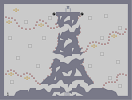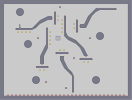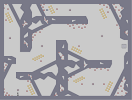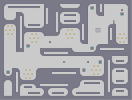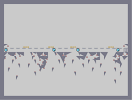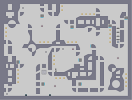Sentry Objects, etc. Webbed Modern Trench Warfare Abstract

Five!

### calvin and hobbes

are unbelivable! we also have them in germany... and they are both great :D 5/5

### Thumbnail and Full-size are incredible

you...are...amazing...

LOOK OUT WORLD!!!

### voted it a 5

was about to make a good comment
then realized it was you jawbit.
nice

### Well

I often find that when I'm demoing on nreality, yours is the one I have to work hard to beat :P

### You're a pretty good player.

Interested? [forum.therealn.com]

### it's just a start

11111111111111111111111114000000000000000003111214000000000000000315110214000000000000031501100214000000000003150011000214000000000315000110000214000000031500001100000210000000150000011000000000000000000000110000000000000000000001100000000000000000000011000000000010000000000110000000000100000000001100000000111110000000011000000001010100000000110000001111111110000001100000000101010000000011000000001111100000000110000000000100000000001100000000001000000000011000000000000000000000110000000000000000000001100000000000000000000011000003100000001400000110000315000000021400001100031500000000021400011003150000000000021400110315000000000000021401131500000000000000021411150000000000000000021111111111111111111111111|

### 5/5

Haha, it's perfect!

### is that how long this was up there?

I was asleep! Awesome!

### 14 hours

very impressive jawbit =D

### you

could have put the hair in yellow using gold. it would be better anyways 5/5:)

:D

### wow

this has been on the hot maps page for 7 hours

faved.

### This was well done.

Plus Calvin and Hobbes <3

### WOW

still on the hot maps page. 4 hours later.

### It's quite messy.

It looks great in the thumb but not so much in th full view.
I love Calvin and Hobbes though.

3

### sorry

but I dont agree with everyone... this is not 5 worthy...
good but not that good.
\$3

### haha

just think how long charizard took =P

about this one. calvin and hobbes is one of the best. I put it right up there with charlie brown and garfield (in fact, i dont really care for any others ;D)

but since i dont rate based on how much i like the topic of choice (as i wish others would do *wink, wink*) i have to say... 5/5!!!!

awesome choice, amazing execution. actually 5aved.

5

### well Calvin and Hobbes has been my...

favorite cartoon ever since I was a kid so I have no choice but to 5ave this. That being said I also want to make clear that this is very well done.

this is swell.
what D6 said.

### Excellent sir,

especially your attention to line thickness

5aved

### much

appreciated, I think it got sniped earlier
nice

I can tell

### great

n-art
I LOVE CALVIN AND HOBBES
=D
5aved

5/5
Sweet :P

5aved

### Calvin and Hobes N-art

> (is greater than) epic win

:3

### WOW!!

Awesome! 5/5. glad to see someone remembering the good ol' comics! And what a good way to do so!
cool